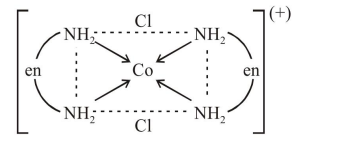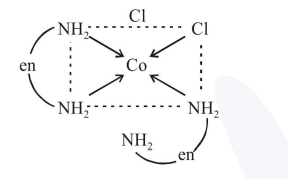# Consider the complex ions,

Question:

Consider the complex ions,

$\operatorname{trans}-\left[\mathrm{Co}(\mathrm{en})_{2} \mathrm{Cl}_{2}\right]^{+}(\mathrm{A})$ and

cis- $\left[\mathrm{Co}(\mathrm{en})_{2} \mathrm{Cl}_{2}\right]^{+}(\mathrm{B})$. The correct statement regarding them is :

1. both (A) and (B) can be optically active

2. both (A) and (B) cannot be optically active

3. (A) can be optically active, but (B) cannot be optically active

4. (A) cannot be optically active, but (B) can be optically active

Correct Option: 4,

Solution:

(A) $\operatorname{trans}-\left[\mathrm{Co}(\mathrm{en})_{2} \mathrm{Cl}_{2}\right]^{+}$$\Rightarrow$ (A) is trans form and shows plane of symmetry which is optically inactive (not optically active)

(B) cis- $\left[\mathrm{Co}(\mathrm{en})_{2} \mathrm{Cl}_{2}\right]^{+}$$\Rightarrow$ (B) is cis form and does not shows plane of symmetry, hence it is optically active.